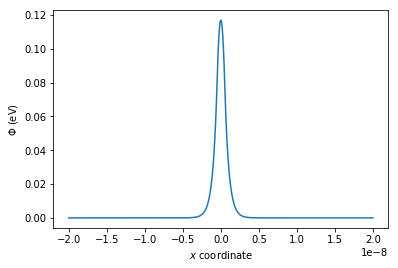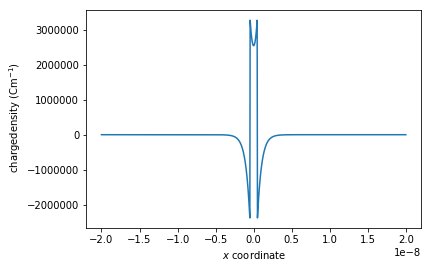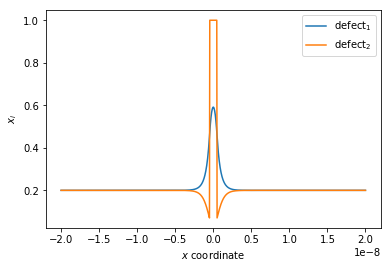## Running the calculation¶

Running the code under Gouy-Chapman conditions takes approximately 19 minutes (iMac with 4 Ghz i7 processor).

This is due to the Poisson-Boltzmann solver being run multiple times to minimise the difference between input and output mole fractions.

In :
from pyscses.defect_species import DefectSpecies
from pyscses.set_of_sites import Set_of_Sites
from pyscses.constants import boltzmann_eV
from pyscses.calculation import Calculation, calculate_activation_energies
from pyscses.set_up_calculation import calculate_grid_offsets
from pyscses.grid import Grid

import numpy as np
import pandas as pd
import matplotlib.pyplot as plt
%matplotlib inline

In :
boundary_conditions = 'dirichlet'
site_charges = False
systems = 'gouy-chapman'
core_models = False
site_models = 'continuum'

In :
alpha = 0.0005

conv = 1e-8
grid_x_min = -2.0e-8
grid_x_max = +2.0e-8
bulk_x_min = -2.0e-8
bulk_x_max = -1.0e-8

dielectric = 1

index = 111

b = 5e-9
c = 5e-9

temp = [773.15]

In :
valence = [ +1.0, -1.0 ]
site_labels = ['site_1', 'site_2']
defect_labels = ['defect_1', 'defect_2']
mole_fractions = [ [ 0.2, 0.2 ] ]
initial_guess = [ [ 0.2, 0.2 ] ]
mobilities = [1.0, 1.0]

In :
data = '../input_data/example_data_2_one_seg_energies.txt'


The limits are the widths between the midpoint of the final site and an 'imaginary' site extending beyond the calculation unit. These are calculated to use in delta_x calculations where the width of each site is required to calculate volumes or densities. The laplacian_limits are the widths between the end sites.

limits, laplacian_limits = calculate_grid_offsets( data, grid_x_min, grid_x_max, 'single' )

If the calculation is being run over a number of different conditions, the mole fractions are looped over.

for m in mole_fractions:

If the calculation is being run over a number of different conditions, the temperatures are looped over.

for t in temp:

Next the different defect species are defined using the Defect_Species class. This collects and stores the information about each defect, the defect label, the defect valence and the defect mole fraction.

defect_species = { l : Defect_Species( l, v, m ) for l, v, m in zip( defect_labels, valence, m) }

The input data file and system information are passed into set_of_sites_from_input_data, which formats and splits wach line of the input file to create Site objects for the individual sites, which are then grouped together into a Set_of_Sites object.

all_sites = Set_of_Sites.set_of_sites_from_input_data( data, [grid_x_min, grid_x_max], defect_species, site_charges, core_models, t )

The code checks whether the model being used is continuum, if so, the sites are passed into form_continuum_sites which interpolates the defect segregation energies onto a regular grid.

if site_models == 'continuum':
all_sites, limits = Set_of_Sites.form_continuum_sites( all_sites, grid_x_min, grid_x_max, 1000, b, c, defect_species, laplacian_limits, site_labels, defect_labels )

ace charge models typically consider dopant ions as either mobile or immobile. If the dopant ions are considered immobile, the model follows a Mott-Schottky approximation. If the dopant ions are considered mobile, the model follows a Gouy-Chapman approximation. The code checks whether a Mott-Schottky or Gouy-Chapman approximation is being applied and fixes the mole fractions of the defects accordingly.

if systems == 'mott-schottky':
for site in all_sites.subset( 'site_2' ):
site.defect_with_label('defect_2').fixed = True
if systems == 'gouy-chapman':
for site in all_sites.subset( 'site_2' ):
site.defect_with_label('defect_2').fixed = False

A grid is created using the Grid class. This includes the array of $x$ coordinates, b/c (the width and height of the cell used in the atomistic simulation) and the full set of sites all_sites

grid = Grid.grid_from_set_of_sites( all_sites, limits, laplacian_limits, b, c )

Following this, a Calculation object is created. This class contains the functions which calculate the space charge properties if the system.

c_o = Calculation( grid, bulk_x_min, bulk_x_max, alpha, conv, t, boundary_conditions, initial_guess )

Subgrids are created for each of the defect species.

c_o.form_subgrids( site_labels )

If the system follows Gouy-Chapman conditions, and periodic boundary conditions are being enforced, a mole fraction correction is required to ensure the output bulk mole fraction is equivalent to the input bulk mole fraction. This function runs the Poisson-Boltzmann calculation and minimises the difference between the target and output mole fractions. This is not required when running the calculation using Mott-Schottky conditions.

c_o.mole_fraction_correction( m, system )

Next, the Poisson-Boltzmann equation is solved for the given system. The potential and charge density are calculated. This uses a function calculation which takes as arguments grid, conv, temp, alpha and boundary_conditions. The calculation function creates numpy arrays the same length as the grid including zeros for the potential (phi) and the charge density (rho).

Solving the Poisson-Boltzmann equation

1. The invertible matrix $A$ is created from the distances between each of the points on the grid. Initially the full matrix is created using scipy.sparse.diags and is then converted into a sparse tridiagonal matrix using scipy.sparse.csc_matrix and the calculation follows the finite difference approximation method defined above.
2. The convergence is initialised as 1 and while it is larger than conv defined in the notebook the calculation will iterate.
3. Using the phi array of zeros, the charge density is calculated at each site. Using the calculated value for the charge density at each site $\Phi = A^{-1}b$ is solved using scipy.linalg.spsolve. This returns predicted_phi at each site, which is damped using $\alpha$ to become the new phi array. The convergence is updated using $\frac{ \sum_i ( ( \Phi_{predicted} - \Phi )^2 )}{L_{grid.x}}$ where $L_{grid.x}$ is the length of the grid.
c_o.solve(system)

Using the calculated equilibrium electrostatic potentials at each site, the defect mole fractions can be calculated.

c_o.mole_fractions()

The resistivity ratio can be calculated from the previously calculated defect concentrations. c_o.calculate_resistivity_ratio takes two arguments. Firstly, whether the space charge potential is positive or negative and secondly a limit for the value of the potential that the site must exceed to be considered as the space charge region.

c_o.calculate_resistivity_ratio( 'positive', 2e-2 )

If the calculation is run over a number of temperatures, and the resistivity ratios stored in a list the activation energy can be calculates.

c_o.calculate_activation_energies( resistivity_ratios, temp )
In :
limits, laplacian_limits = calculate_grid_offsets( data, grid_x_min, grid_x_max, 'single' )

for m in mole_fractions:
for t in temp:

defect_species = { l : DefectSpecies( l, v, m, mob ) for l, v, m, mob in zip( defect_labels, valence, m, mobilities ) }

all_sites = Set_of_Sites.set_of_sites_from_input_data( data, [grid_x_min, grid_x_max], defect_species, site_charges, core_models, t )
if site_models == 'continuum':
all_sites, limits = Set_of_Sites.form_continuum_sites( all_sites, grid_x_min, grid_x_max, 1000, b, c, defect_species, laplacian_limits, site_labels, defect_labels )
if systems == 'mott-schottky':
for site in all_sites.subset( 'site_2' ):
site.defect_with_label('defect_2').fixed = True
if systems == 'gouy-chapman':
for site in all_sites.subset( 'site_2' ):
site.defect_with_label('defect_2').fixed = False
grid = Grid.grid_from_set_of_sites( all_sites, limits, laplacian_limits, b, c )

c_o = Calculation( grid, bulk_x_min, bulk_x_max, alpha, conv, dielectric, t, boundary_conditions )
c_o.form_subgrids( site_labels )
if systems == 'gouy-chapman':
c_o.mole_fraction_correction( m, systems, initial_guess )
c_o.solve(systems)
c_o.mole_fractions()
c_o.calculate_resistivity_ratio( 'positive', 2e-2 )

--- 1132.7030682563782 seconds ---


The space charge properties can then be plot, using matplotlib.pyplot.

In :
plt.plot(grid.x, c_o.phi)
plt.xlabel( '$x$ $\mathrm{coordinate}$' )
plt.ylabel('$\Phi$ $\mathrm{( eV )}$')
plt.show()

plt.plot(grid.x, c_o.rho)
plt.xlabel( '$x$ $\mathrm{coordinate}$'  )
plt.ylabel(' $\mathrm{charge density}$ $(\mathrm{C m}^{-1})$')
plt.show()

plt.plot(grid.x, c_o.mf[site_labels], label = '$\mathrm{defect_1}$')
plt.plot(grid.x, c_o.mf[site_labels], label = '$\mathrm{defect_2}$')
plt.xlabel( '$x$ $\mathrm{coordinate}$'  )
plt.ylabel('$x_{i}$')
plt.legend()
plt.show()